When building a machine learning algorithm, such as a regression or classification algorithm, a common goal is to produce a generalized model. This is so that it performs well on new data that the model has not seen before. Overfitting and underfitting are two fundamental causes of poor performance for machine learning models. A model is overfitted when it performs well on known data, but generalizes poorly on new data. However, an underfit model performs poorly on both trained and new data. A reliable model validation technique helps provide better assessment for predicting model performance in practice, and provides insight for training models to achieve the best accuracy.

Cross-validation is a standard model validation technique commonly used for assessing performance of machine learning algorithms. In general, it works by first sampling the dataset into groups of similar sizes, where each group contains a subset of data dedicated for training and model evaluation. After the data has been grouped, a machine learning algorithm will fit and score a model using the data in each group independently. The final score of the model is defined by the average score across all the trained models for performance metric representation.

There are few cross-validation methods commonly used, including k-fold, stratified k-fold, and leave-p-out, to name a few. Although there are well-defined data science frameworks that can help simplify cross-validation processes, such as Python scikit-learn library, these frameworks are designed to work in a monolithic, single compute environment. When it comes to training machine learning algorithms with large volume of data, these frameworks become bottlenecked with limited scalability and reliability.

In this blog post, we are going to walk through the steps for building a highly scalable, high-accuracy, machine learning pipeline, with the k-fold cross-validation method, using Amazon Simple Storage Service (Amazon S3), Amazon SageMaker Pipelines, SageMaker automatic model tuning, and SageMaker training at scale.

## Overview of solution

To operate the k-fold cross validation training pipeline at scale, we built an end to end machine learning pipeline using SageMaker native features. This solution implements the k-fold data processing, model training, and model selection processes as individual components to maximize parallellism. The pipeline is orchestrated through SageMaker Pipelines in distributed manner to achieve scalability and performance efficiency. Let’s dive into the high-level architecture of the solution in the following section.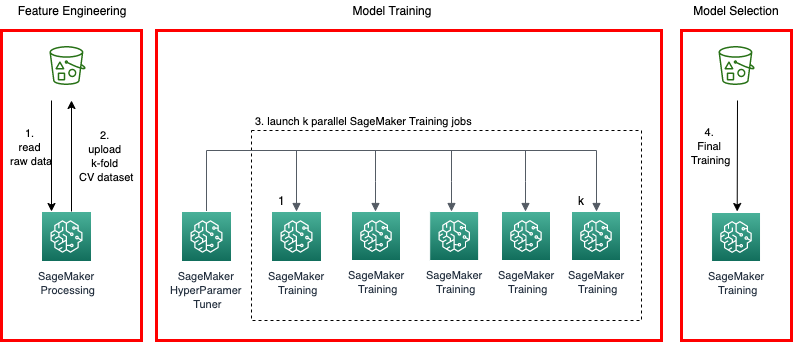Figure 1. Solution architecture

The overall solution architecture is shown in Figure 1. There are four main building blocks in the k-fold cross-validation model pipeline:

1. Preprocessing – Sample and split the entire dataset into k groups.
2. Model training – Fit the SageMaker training jobs in parallel with hyperparameters optimized through the SageMaker automatic model tuning job.
3. Model selection – Fit a final model, using the best hyperparameters obtained in step 2, with the entire dataset.
4. Model registration – Register the final model with SageMaker Model Registry, for model lifecycle management and deployment.

The final output from the pipeline is a model that represents best performance and accuracy for the given dataset. The pipeline can be orchestrated easily using a workflow management tool, such as Pipelines.

Amazon SageMaker is a fully managed service that enables data scientists and developers to quickly develop, train, tune, and deploy machine learning quickly and at scale. When it comes to choosing the right machine learning and data processing frameworks to solve problems, SageMaker gives you the flexibility to use prebuilt containers bundled with the supported common machine learning frameworks—such as Tensorflow, Pytorch, and MxNet—or to bring your own container images with custom scripts and libraries that fit your use cases to train on the highly available SageMaker model training environment. Additionally, Pipelines enables users to develop complete machine learning workflows using python SDK, and manage these workflows in SageMaker Studio.

For simplicity, we will use the public Iris flower data as the train and test dataset to build a multivariate classification model using linear algorithm (SVM). The pipeline architecture is agnostic to the data and model; hence, it can be modified to adopt a different dataset or algorithm.

## Prerequisites

To deploy the solution, you require the following:

• SageMaker Studio
• A Command Line (Terminal) that supports building Docker images (or instance, AWS Cloud9)

## Solution walkthrough

In this section, we are going to walk through the steps to create a cross-validation model training pipeline using Pipelines. The main components are as follows.

1. Pipeline parameters
Pipelines parameters are introduced as variables that allow the predefined values to be overridden at runtime. Pipelines supports the following parameters types: String, Integer, and Float (expressed as ParameterString, ParameterInteger, and ParameterFloat). The following are some examples of the parameters used in the cross-validation model training pipeline:
• K-Fold – Value of k to be used in k-fold cross-validation
• ProcessingInstanceCount – Number of instances for SageMaker processing job
• ProcessingInstanceType – Instance type used for SageMaker processing job
• TrainingInstanceType – Instance type used for SageMaker training job
• TrainingInstanceCount – Number of instances for SageMaker training job
1. Preprocessing

In this step, the original dataset is split into k equal-sized samples. One of the k samples is retained as the validation data for model evaluation, with the remaining k-1 samples to be used as training data. This process is repeated k times, with each of the k samples used as the validation set only one time. The k sample collections are uploaded to an S3 bucket, with the prefix corresponding to an index (0 – k-1) to be identified as the input path to the specified training jobs in the next step of the pipeline. The cross-validation split is submitted as a SageMaker processing job orchestrated through the Pipelines processing step. The processing flow is shown in Figure 2.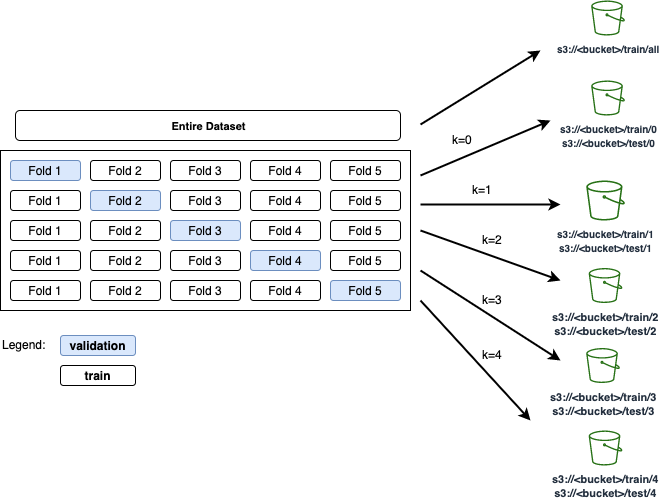Figure 2. K-fold cross-validation: original data is split into k equal-sized samples uploaded to S3 bucket

The following code snippet splits the k-fold dataset in the preprocessing script:

``def save_kfold_datasets(X, y, k): """ Splits the datasets (X,y) k folds and saves the output from each fold into separate directories. Args: X : numpy array represents the features y : numpy array represetns the target k : int value represents the number of folds to split the given datasets """ # Shuffles and Split dataset into k folds. kf = KFold(n_splits=k, random_state=23, shuffle=True) fold_idx = 0 for train_index, test_index in kf.split(X, y=y, groups=None): X_train, X_test = X[train_index], X[test_index] y_train, y_test = y[train_index], y[test_index] os.makedirs(f'{base_dir}/train/{fold_idx}', exist_ok=True) np.savetxt(f'{base_dir}/train/{fold_idx}/train_x.csv', X_train, delimiter=',') np.savetxt(f'{base_dir}/train/{fold_idx}/train_y.csv', y_train, delimiter=',') os.makedirs(f'{base_dir}/test/{fold_idx}', exist_ok=True) np.savetxt(f'{base_dir}/test/{fold_idx}/test_x.csv', X_test, delimiter=',') np.savetxt(f'{base_dir}/test/{fold_idx}/test_y.csv', y_test, delimiter=',') fold_idx += 1``
1.  Cross-validation training with SageMaker automatic model tuning

In a typical cross-validation training scenario, a chosen algorithm is trained for k times with specific training and a validation dataset sampled through the k-fold technique, mentioned in the previous step. Traditionally, the cross-validation model training process is performed sequentially on the same server. This method is inefficient and doesn’t scale well for models with large volumes of data. Because all the samples are uploaded to an S3 bucket, we can now run k training jobs in parallel. Each training job will consume input samples in the specified bucket location correspond to the index (ranged between 0 – k-1) given to the training job. Additionally, the hyperparameter values must be the same for all k jobs because cross validation estimates the true out-of-sample performance of a model trained with this specific set of hyperparameters.

Although the cross-validation technique helps generalize the models, hyperparameter tuning for the model is typically performed manually. In this blog post, we are going to take a heuristic approach of finding the most optimized hyperparameters using SageMaker automatic model tuning.

We start by defining a training script that accepts the hyperparameters as input for the specified model algorithm, and then implement the model training and evaluation steps.

The steps involved in the training script are summarized as follows:

1. Parse hyperparameters from the input.
2. Fit the model using the parsed hyperparameters.
3. Evaluate model performance (score).
4. Save the trained model.
``if __name__ == '__main__': parser = argparse.ArgumentParser() parser.add_argument('-c', '--c', type=float, default=1.0) parser.add_argument('--gamma', type=float) parser.add_argument('--kernel', type=str) # Sagemaker specific arguments. Defaults are set in the environment variables. parser.add_argument('--output-data-dir', type=str, default=os.environ['SM_OUTPUT_DATA_DIR']) parser.add_argument('--model-dir', type=str, default=os.environ['SM_MODEL_DIR']) parser.add_argument('--train', type=str, default=os.environ['SM_CHANNEL_TRAIN']) parser.add_argument('--test', type=str, default=os.environ.get('SM_CHANNEL_TEST')) args = parser.parse_args() model = train(train=args.train, test=args.test) evaluate(test=args.test, model=model) dump(model, os.path.join(args.model_dir, "model.joblib"))``

Next, we create a python script that performs cross-validation model training by submitting k SageMaker training jobs in parallel with given hyperparameters. Additionally, the script monitors the progress of the training jobs, and calculates the objective metrics by averaging the scores across the completed jobs.

Now we create a python script that uses a SageMaker automatic model tuning job to find the optimal hyperparameters for the trained models. The hyperparameter tuner works by running a specified number of training jobs using the ranges of hyperparameters specified. The number of training jobs and ranges of hyperparameters are given in the input parameter to the script. After the tuning job completes, the objective metrics, as well as the hyperparameters from the best cross-validation model training job, are captured, formatted in JSON format, respectively, to be used in the next steps of the workflow. Figure 3 illustrates cross-validation training with automatic model tuning.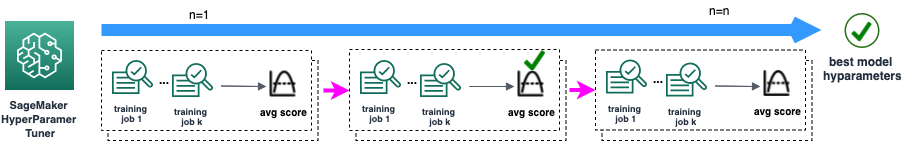Figure 3. In cross-validation training step, a SageMaker HyperparameterTuner job invokes n training jobs. The metrics and hyperparameters are captured for downstream processes.

Finally, the training and cross-validation scripts are packaged and built as a custom container image, available for the SageMaker automatic model tuning job for submission. The following code snippet is for building the custom image:

``````FROM python:3.7
RUN apt-get update && pip install sagemaker boto3 numpy sagemaker-training
COPY cv.py /opt/ml/code/train.py
COPY scikit_learn_iris.py /opt/ml/code/scikit_learn_iris.py
ENV SAGEMAKER_PROGRAM train.py``````
1. Model evaluation
The objective metrics in the cross-validation training and tuning steps define the model quality. To evaluate the model performance, we created a conditional step that compares the metrics against a baseline to determine the next step in the workflow. The following code snippet illustrates the conditional step in detail. Specifically, this step first extracts the objective metrics based on the evaluation report uploaded in previous step, and then compares the value with baseline_model_objective_value provided in the pipeline job. The workflow continues if the model objective metric is greater than or equal to the baseline value, and stops otherwise.
``````from sagemaker.workflow.conditions import ConditionGreaterThanOrEqualTo
from sagemaker.workflow.condition_step import ( ConditionStep, JsonGet,
)
cond_gte = ConditionGreaterThanOrEqualTo( left=JsonGet( step=step_cv_train_hpo, property_file=evaluation_report, json_path="multiclass_classification_metrics.accuracy.value", ), right=baseline_model_objective_value,
)
step_cond = ConditionStep( name="ModelEvaluationStep", conditions=[cond_gte], if_steps=[step_model_selection, step_register_model], else_steps=[],
)``````
1. Model Selection
At this stage of the pipeline, we’ve completed cross-validation and hyperparameter optimization steps to identify the best performing model trained with the specific hyperparameter values. In this step, we are going to fit a model using the same algorithm used in cross-validation training by providing the entire dataset and the hyperparameters from the best model. The trained model will be used for serving predictions for downstream applications. The following code snippet illustrates a Pipelines training step for model selection:
``````from sagemaker.inputs import TrainingInput
from sagemaker.workflow.steps import TrainingStep
from sagemaker.sklearn.estimator import SKLearn
sklearn_estimator = SKLearn("scikit_learn_iris.py", framework_version=framework_version, instance_type=training_instance_type, py_version='py3', source_dir="code", output_path=s3_bucket_base_path_output, role=role)
step_model_selection = TrainingStep( name="ModelSelectionStep", estimator=sklearn_estimator, inputs={ "train": TrainingInput( s3_data=f'{step_process.arguments["ProcessingOutputConfig"]["Outputs"]["S3Output"]["S3Uri"]}/all', content_type="text/csv" ), "jobinfo": TrainingInput( s3_data=f"{s3_bucket_base_path_jobinfo}", content_type="application/json" ) }
)``````
1. Model registration
Because the cross-validation model training pipeline evolves, it’s important to have a mechanism for managing the version of model artifacts over time, so that the team responsible for the project can manage the model lifecycle, including track, deploy, or rollback a model based on the version. Building your own model registry, with lifecycle management capabilities, can be complicated and challenging to maintain and operate. SageMaker Model Registry simplifies model lifecycle management by enabling model catalog, versioning, metrics association, model approval workflow, and model deployment automation.

In the final step of the pipeline, we are going to register the trained model with Model Registry by associating model objective metrics, the model artifact location on S3 bucket, the estimator object used in the model selection step, model training and inference metadata, and approval status. The following code snippet illustrates the model registry step using ModelMetrics and RegisterModel.

``````from sagemaker.model_metrics import MetricsSource, ModelMetrics
from sagemaker.workflow.step_collections import RegisterModel
model_metrics = ModelMetrics( model_statistics=MetricsSource( s3_uri="{}/evaluation.json".format( step_cv_train_hpo.arguments["ProcessingOutputConfig"]["Outputs"]["S3Output"]["S3Uri"] ), content_type="application/json", )
)
step_register_model = RegisterModel( name="RegisterModelStep", estimator=sklearn_estimator, model_data=step_model_selection.properties.ModelArtifacts.S3ModelArtifacts, content_types=["text/csv"], response_types=["text/csv"], inference_instances=["ml.t2.medium", "ml.m5.xlarge"], transform_instances=["ml.m5.xlarge"], model_package_group_name=model_package_group_name, approval_status=model_approval_status, model_metrics=model_metrics,``````

Figure 4 shows a model version registered in SageMaker Model Registry upon a successful pipeline job through Studio.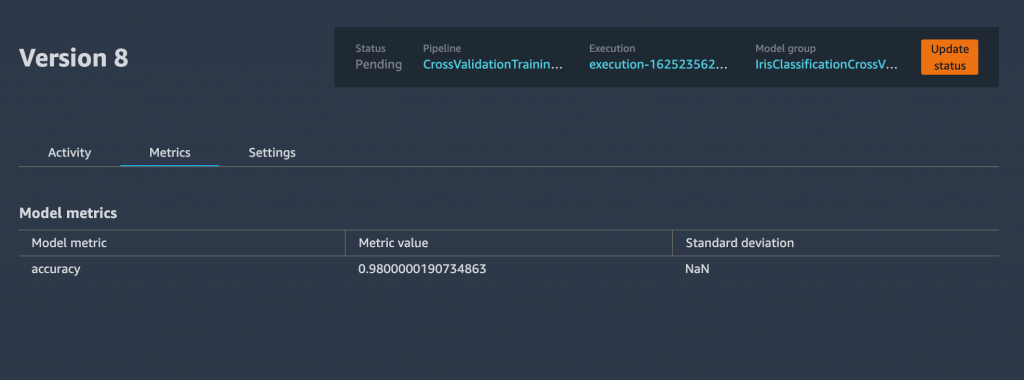Figure 4. Model version registered successfully in SageMaker

1. Putting everything together
Now that we’ve defined a cross-validation training pipeline, we can track, visualize, and manage the pipeline job directly from within Studio. The following code snippet and Figure 5 depicts our pipeline definition:
``````from sagemaker.workflow.pipeline_experiment_config import PipelineExperimentConfig
from sagemaker.workflow.execution_variables import ExecutionVariables
pipeline_name = f"CrossValidationTrainingPipeline"
pipeline = Pipeline( name=pipeline_name, parameters=[ processing_instance_count, processing_instance_type, training_instance_type, training_instance_count, inference_instance_type, hpo_tuner_instance_type, model_approval_status, role, default_bucket, baseline_model_objective_value, bucket_prefix, image_uri, k, max_jobs, max_parallel_jobs, min_c, max_c, min_gamma, max_gamma, gamma_scaling_type ], pipeline_experiment_config=PipelineExperimentConfig( ExecutionVariables.PIPELINE_NAME, ExecutionVariables.PIPELINE_EXECUTION_ID), steps=[step_process, step_cv_train_hpo, step_cond],``````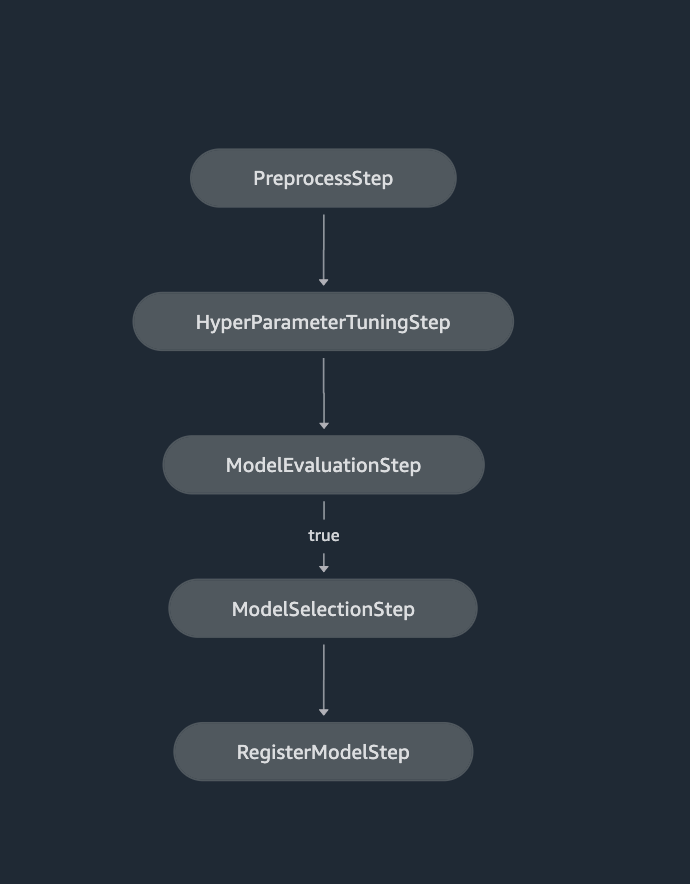Figure 5. SageMaker Pipelines definition shown in SageMaker Studio

Finally, to kick off the pipeline, invoke the pipeline.start() function, with optional parameters specific to the job run:

``execution = pipeline.start( parameters=dict( BaselineModelObjectiveValue=0.8, MinimumC=0, MaximumC=1 ))``

You can track the pipeline job from within Studio, or use SageMaker application programming interfaces (APIs). Figure 6 shows a screenshot of a pipeline job in progress from Studio.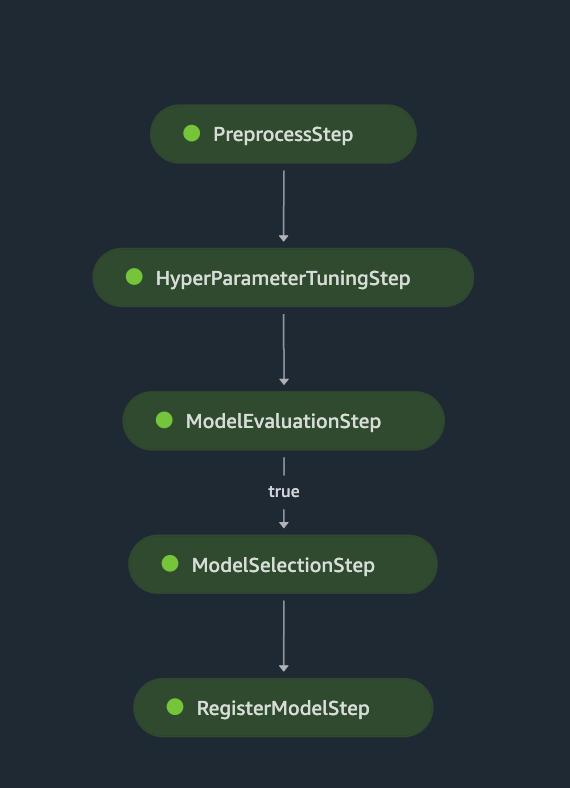Figure 6. SageMaker Pipelines job progress shown in SageMaker Studio

## Conclusion

In this blog post, we showed you an architecture that orchestrates a complete workflow for cross-validation model training. We implemented the workflow using SageMaker Pipelines that incorporates preprocessing, hyperparameter tuning, model evaluation, model selection, and model registration. The solution addresses the common challenge of orchestrating cross-validation model pipeline at scale. The entire pipeline implementation, including a jupyter notebook that defines the pipeline, a Dockerfile and python scripts described in this blog post, can be found in the GitHub project.

##### Field Notes provides hands-on technical guidance from AWS Solutions Architects, consultants, and technical account managers, based on their experiences in the field solving real-world business problems for customers.
Categories: Architecture